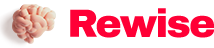# How to Reduce ESL (Equivalent Series Inductance) of Decoupling Capacitors

SaveSavedRemoved 0
Deal Score0
Deal Score0

The second point of effective use of decoupling capacitors is to reduce the capacitor’s ESL (ie, equivalent series inductance). Although it is said to “reduce ESL”, since the ESL itself of a single product cannot be changed, it means “even if the capacitance value is the same, use a capacitor with a small ESL”. By lowering the ESL, high-frequency characteristics can be improved, and high-frequency noise can be reduced more effectively.

The second point of effective use of decoupling capacitors is to reduce the capacitor’s ESL (ie, equivalent series inductance). Although it is said to “reduce ESL”, since the ESL itself of a single product cannot be changed, it means “even if the capacitance value is the same, use a capacitor with a small ESL”. By lowering the ESL, high-frequency characteristics can be improved, and high-frequency noise can be reduced more effectively.

Use smaller capacitors even with the same capacitance

For multilayer ceramic capacitors (MLCCs), several packages with the same capacitance value but different sizes are sometimes prepared. ESL depends on the structure of the pin area. Capacitors with smaller sizes basically have smaller lead parts, and usually have smaller ESLs.

The figure on the right is an example of the frequency characteristics of capacitors with the same capacitance but different sizes. As shown, the smaller 1005 size has a higher resonant frequency and a lower impedance in the frequency range after the inductive region. As described in “Frequency Characteristics of Capacitors”, the resonant frequency of capacitors is based on the following formula. It can be seen from the formula that as long as the capacitance values ​​are the same, the lower the ESL, the higher the resonant frequency. In addition, the impedance characteristic of the inductive region depends on the ESL, which was also described.Regarding noise countermeasures, when it is necessary to reduce noise in higher frequency bands, small-sized capacitors can be selected.

Use capacitors designed to reduce ESL

Among MLCCs, there are some models with shapes and structures designed to reduce ESL.

As shown in the figure, the electrodes of ordinary capacitors are on the short side, while the electrodes of the LW inversion type are opposite, on the long side. Since L (length) and W (width) are opposite, it is called “LW reversal type”. It is a type of reducing ESL by increasing the width of the electrodes.

The three-terminal capacitor is a capacitor with an optimized structure in order to improve the frequency characteristics of ordinary capacitors (two pins). The three-terminal capacitor is to extend the other end of one pin (electrode) of the two-pin capacitor as a through pin, and use the other pin as a GND pin. In the above figure, the input and output electrodes are equivalent to the through pins extending from both ends, and the left and right electrodes are of course conductive. There is a dielectric between the input and output electrodes (thru pins) and the GND electrode, which acts as a capacitor.

Insert the input and output electrodes into the power supply or signal line in series (connect one end of the input and output electrodes to the input end and the other end to the output end), and the GND electrode is grounded. In this way, since the ESL of the input and output electrodes is not included in the ground terminal, the impedance of the ground becomes very low. In addition, the ESL of the input and output electrodes is directly inserted in the noise path, which is beneficial to reduce noise (increase insertion loss).

ESL can be suppressed by arranging GND electrodes in pairs on the long side, and ESL can be halved by connecting them in parallel.

Based on this structure, the three-terminal capacitor not only has very low ESL, but also maintains low ESR, which can significantly improve high-frequency characteristics compared with two-pin capacitors with the same capacitance and the same size.

Key takeaways:

• There are two main points for the effective use of decoupling capacitors: ① Use multiple capacitors, ② Reduce the ESL of the capacitors.
• By reducing the ESL of the capacitor, high frequency characteristics can be improved and high frequency noise can be reduced more effectively.
• Some capacitors have the same capacitance, but have a smaller ESL due to their size and structure.